Latest Banking jobs   »   Reasoning Ability Quiz For SBI Clerk...

# Reasoning Ability Quiz For SBI Clerk Prelims 2021- 3rd June

Directions (1-3): Study the following information carefully and answer the questions given below:
Point B is in 12m north of point A. Point B is 10m in west of point C. Point C is 20m north of point D. Point D is 15m east of Point E. Point F is 10m north of point E. Point G is 5m east of point F.

Q1. If a person starts walking from point G, then at which point he will reach first?
(a) E
(b) A
(c) F
(d) B
(e) C

Q2. If Point U is 8m south of point A then what will be the distance between point U and E?
(a) 9m
(b) 5m
(c) 4m
(d) 6m
(e) 7m

Q3. Point C is in which direction from point E?
(a) South
(b) South-east
(c) North
(d) North-east
(e) North-west

Direction (4-6): Study the following information to answer the given questions:
Point G is 10m north of point A. Point B is 10m east of point A. Point C is 20m south of point B. Point D is 10m west of point C. Point E is 10m north of point D. Point F is 15m west of point E. Point H is 15m north of point F. Point K is 5m east of point H.

Q4. In which direction is point K with respect to point G?
(a) South-west
(b) North-east
(c) North-west
(d) South-east
(e) None of these

Q5. If point Z is 10m east of point K, then point A is how far from point Z?
(a) 10m
(b) 5m
(c) 15m
(d) 20m
(e) None of these

Q6. If point J is exactly midpoint of BC then point E is how far from point J?
(a) 10m
(b) 5m
(c) 15m
(d) 20m
(e) None of these

Directions (7-9): Study the information carefully and answer the questions given below.
A is 4m south to B. D is 5m west to E. H is 4m south to G. C is 8m north to D. C is 4m east B. G is 3m east to F. E is 12m south to F. I is mid-point of F and E.

Q7. In which direction is E with respect to C?
(a) South-East
(b) South-West
(c) North-East
(d) North-West
(e) None of these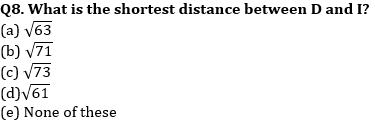Q9. In which direction is point A with respect to G?
(a) South-east
(b) South-west
(c) North-east
(d) North-west
(e) None of these

Directions (10-12): Study the information carefully and answer the questions given below:
Vertical axis is running from H to I, which is north to H. Horizontal axis runs from G to F, which is east to G. B is midpoint of both the axis. A is 1m north to G. E is 1m west to H. D is 1m south to F. C is 1 m east to I. Length to GF and HI is same i.e. 2m.

Q10. In which direction is point G with respect to C?
(a) North-east
(b) North-west
(c) South-east
(d) South-west
(e) None of these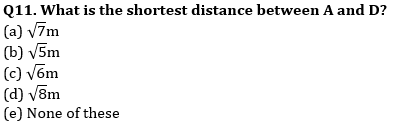Q12. In which direction is point I with respect to F?
(a) North-east
(b) North-west
(c) South-east
(d) South-west
(e) None of these

Directions (13-15): Study the information carefully and answer the questions given below.
A walks for 4m from point X in the east direction. He then takes a right and a left turn and walked for 3m and 2m respectively and finally reaches point Z. B starts walking from point Y and walks for 8m in east direction, he then takes three consecutive right turns and walks 10m, 2m and 3m respectively and reaches Z.

Q13. In which direction is point Z with respect to point Y?
(a) South-west
(b) North-east
(c) South-east
(d) North-west
(e) None of these

Q14. What is shortest distance between point X and point Y?
(a) 10m
(b) 6m
(c) 8m
(d) 4m
(e) None of these

Q15. In which direction is point X with respect to point Y?
(a) North
(b) East
(c) South
(d) West
(e) None of these

Practice More Questions of Reasoning for Competitive Exams:

###### SBI Clerk Study Plan 2021

Solutions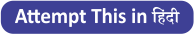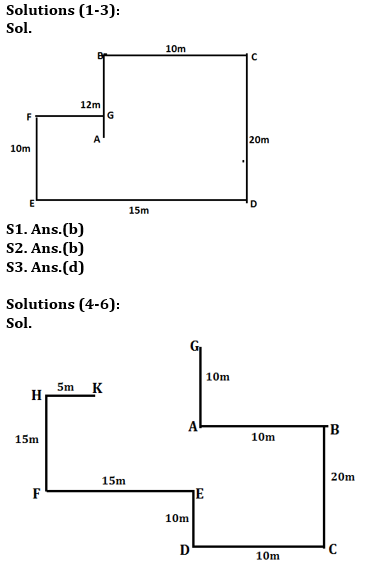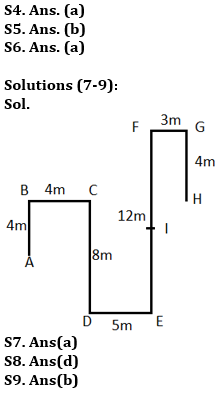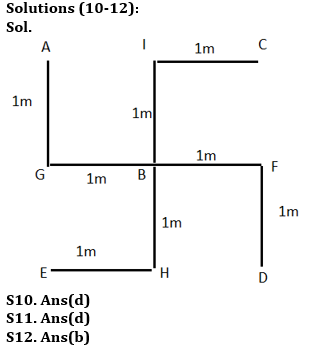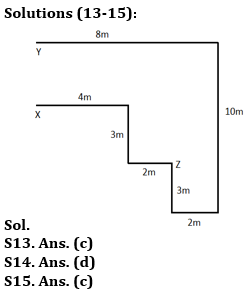#### Congratulations!

Incorrect details? Fill the form again here

•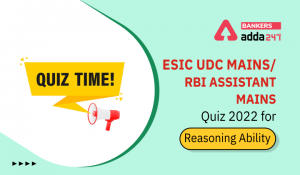Reasoning Ability Quiz For RBI Assistant...
•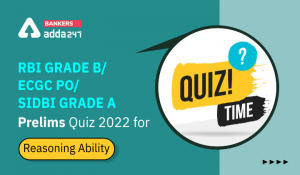Reasoning Ability Quiz For RBI Grade B/ ...
•Reasoning Ability Quiz For RBI Assistant...
•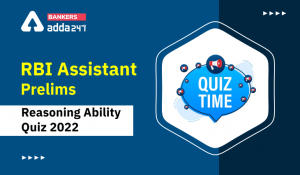Reasoning Ability Quiz For RBI Assistant...
•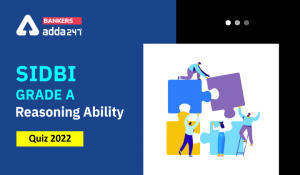Reasoning Ability Quiz For SIDBI GRADE A...
•Reasoning Ability Quiz For RBI Assistant...